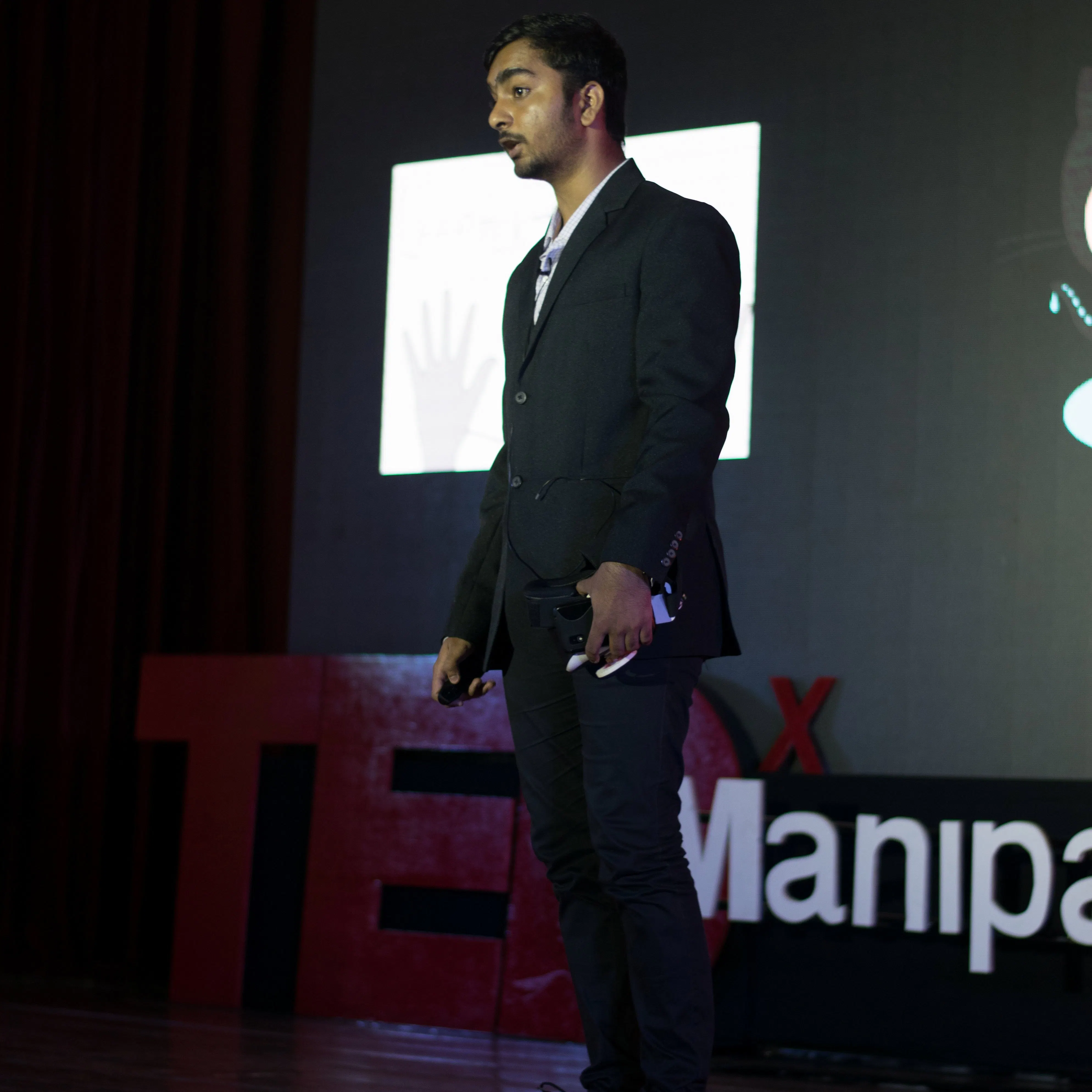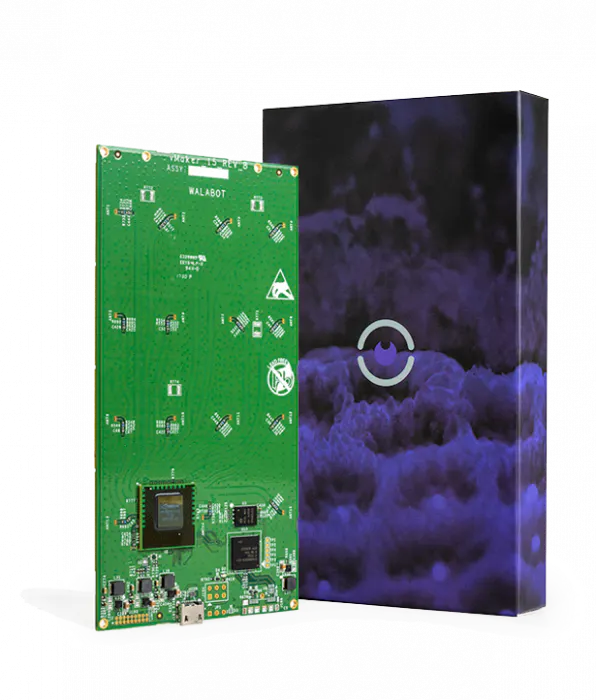# WalaBreathe - A Wireless Breath To Speech Assistive Device

WalaBreathe is a completely wireless assistive device for paraplegics that converts their breath into audible speech built on Python.## Things used in this project

### Hardware componentsWalabot Creator
×1

## Code

### WalaBreathe Source Code

Python
The main parts of the code are explained in the documentation, if you have any other doubts let me know in the comments below or hit me up on hackster messages.
```#    WalaBreathe : A Wireless Breath to Speech Assistive Device for users with motor neuron related disabilities
#    Developer : Geeve George

from __future__ import print_function
from sys import platform
from os import system
from os.path import join

import matplotlib.pyplot as plt #pyplot library for plotting breath 'energy' in real-time
import numpy as np

import time
import array
import os #for e-speak

plt.axis([0, 1000, 0, 100]) #pyplot function for mentioning minimum and maximum 'x' and 'y' axis values
plt.ion() #enables interactive plotting

counter = 0
b_magnitude = 0
b_counter = 0
nb_counter = 0
gen_counter = 0
lb_counter = 0
sb_counter = 0
i = 0
bn_counter = 0    #Breath Stop Detection

msg_array = []    #an unsized list created to store 'dots' & 'dashes'
wrd_array = []    #an unsized list created to store english alphabets

list_counter = 0    #a counter created to keep track of list input index

msg_counter = 0
wrd_counter = 0

if platform == 'win32':
modulePath = join('C:/', 'Program Files', 'Walabot', 'WalabotSDK',
'python', 'WalabotAPI.py')
elif platform.startswith('linux'):
modulePath = join('/usr', 'share', 'walabot', 'python', 'WalabotAPI.py')

wlbt.Init()

def findexact(msg_array,morse):    #a function that checks if content of msg_array and morse are an exact match
i=0
while i < len(msg_array):
if any(item == msg_array[i] for item in morse):
return 1
i+=1

def findexactword(wrd_array,word):    #a function that checks if content of wrd_array and word are an exact match
j=0
#print('Inside Find Exact Word')
while j < len(wrd_array):
if any(items == wrd_array[j] for items in word):
return 1
j+=1

#functions to clear msg & word lists
def clear_ar():
del msg_array[:]

def clear_wrdar():
del wrd_array[:]

def insert_wrd(wrd_array,letter):    #a function that concatinates word input

food = ['FD']
hay = ['HAY']
iaf = ['IAF']
wt = ['WT']
global wrd_counter
if(wrd_counter==0):
wrd_array.append(letter)

else:
wrd_array = [x + letter for x in wrd_array]
wrd_counter+=1

#Short command checking by giving a function call to 'findexactword()' , if returns true then: text to speech output using 'e-speak'

if (findexactword(wrd_array,food)==1):
print('I want food')
os.system("espeak 'I want food'")
wrd_counter=0
clear_wrdar()

print('Word : ')
print(wrd_array)

elif (findexactword(wrd_array,hay)==1):
print('How are you?')
os.system("espeak 'How are you'")
wrd_counter=0
clear_wrdar()

elif (findexactword(wrd_array,iaf)==1):
print('I am fine')
os.system("espeak 'I am fine'")
wrd_counter=0
clear_wrdar()

elif (findexactword(wrd_array,wt)==1):
print('I want water')
os.system("espeak 'I want water'")
wrd_counter=0
clear_wrdar()

def Walabreathe_main(energy):    #the main function in walabreathe where the breath detection,conversion to morse code and speech output takes place

global counter
global b_magnitude
global b_counter
global nb_counter
global gen_counter
global lb_counter
global sb_counter
global msg_array
global msg_counter
global bn_counter
global list_counter

dash = '-'
dot = '.'
m_a = ['.-']
m_b = ['-...']
m_c = ['-.-.']
m_d = ['-..']
m_e = ['.']
m_f = ['..-.']
m_g = ['--.']
m_h = ['....']
m_i = ['..']
m_j = ['.---']
m_k = ['-.-']
m_l = ['.-..']
m_m = ['--']
m_n = ['-.']
m_o = ['---']
m_p = ['.--.']
m_q = ['--.-']
m_r = ['.-.']
m_s = ['...']
m_t = ['-']
m_u = ['..-']
m_v = ['...-']
m_w = ['.--']
m_x = ['-..-']
m_y = ['-.--']
m_z = ['--..']
l_a = 'A'
l_b = 'B'
l_c = 'C'
l_d = 'D'
l_e = 'E'
l_f = 'F'
l_g = 'G'
l_h = 'H'
l_i = 'I'
l_j = 'J'
l_k = 'K'
l_l = 'L'
l_m = 'M'
l_n = 'N'
l_o = 'O'
l_p = 'P'
l_q = 'Q'
l_r = 'R'
l_s = 'S'
l_t = 'T'
l_u = 'U'
l_v = 'V'
l_w = 'W'
l_x = 'X'
l_y = 'Y'
l_z = 'Z'

system('cls' if platform == 'win32' else 'clear')
b_magnitude = energy*1000

counter+=1
gen_counter+=1
if(gen_counter==1):    #prompting user to breathe after previous breath has ended
print('Breathe Now')

plt.scatter(counter,energy*1000)    #pyplot function that allows us to scatter plot breathing 'energy' values
plt.pause(0.005)

if b_magnitude>3:    #user is breathing

b_counter+=1

else:    #not breathing

nb_counter+=1

if(lb_counter+sb_counter>=5 or bn_counter>=2):    #when the morse inputs add up to 5 or the user stops breathing for 3 attempts then morse code checking and conversion takes place

print(msg_array)

if (findexact(msg_array,m_a)==1):    #checking for morse combination match and if true, text to speech speaks the correponding english alphabet

print('A')
os.system("espeak 'A'")
insert_wrd(wrd_array,l_a)

elif (findexact(msg_array,m_b)==1):
print('B')
os.system("espeak 'B'")
#insert_wrd(wrd_array,l_b)

elif (findexact(msg_array,m_c)==1):
print('C')
os.system("espeak 'C'")
#insert_wrd(wrd_array,l_c)

elif (findexact(msg_array,m_d)==1):
print('D')
os.system("espeak 'D'")
insert_wrd(wrd_array,l_d)

elif (findexact(msg_array,m_e)==1):
print('E')
os.system("espeak 'E'")
#insert_wrd(wrd_array,l_e)

elif (findexact(msg_array,m_f)==1):
print('F')
os.system("espeak 'F'")
insert_wrd(wrd_array,l_f)

elif (findexact(msg_array,m_g)==1):
print('G')
os.system("espeak 'G'")
#insert_wrd(wrd_array,l_g)

elif (findexact(msg_array,m_h)==1):
print('H')
os.system("espeak 'H'")
insert_wrd(wrd_array,l_h)

elif (findexact(msg_array,m_i)==1):
print('I')
os.system("espeak 'I'")
insert_wrd(wrd_array,l_i)

elif (findexact(msg_array,m_j)==1):
print('J')
os.system("espeak 'J'")
#insert_wrd(wrd_array,l_j)

elif (findexact(msg_array,m_k)==1):
print('K')
os.system("espeak 'K'")
#insert_wrd(wrd_array,l_k)

elif (findexact(msg_array,m_l)==1):
print('L')
os.system("espeak 'L'")
#insert_wrd(wrd_array,l_l)

elif (findexact(msg_array,m_m)==1):
print('M')
os.system("espeak 'M'")
#insert_wrd(wrd_array,l_m)

elif (findexact(msg_array,m_n)==1):
print('N')
os.system("espeak 'N'")
#insert_wrd(wrd_array,l_n)

elif (findexact(msg_array,m_o)==1):
print('O')
os.system("espeak 'O'")
#insert_wrd(wrd_array,l_o)

elif (findexact(msg_array,m_p)==1):
print('P')
os.system("espeak 'P'")
#insert_wrd(wrd_array,l_p)

elif (findexact(msg_array,m_q)==1):
print('Q')
os.system("espeak 'Q'")
#insert_wrd(wrd_array,l_q)

elif (findexact(msg_array,m_r)==1):
print('R')
os.system("espeak 'R'")
#insert_wrd(wrd_array,l_r)

elif (findexact(msg_array,m_s)==1):
print('S')
os.system("espeak 'S'")
#insert_wrd(wrd_array,l_s)

elif (findexact(msg_array,m_t)==1):
print('T')
os.system("espeak 'T'")
insert_wrd(wrd_array,l_t)

elif (findexact(msg_array,m_u)==1):
print('U')
os.system("espeak 'U'")
#insert_wrd(wrd_array,l_u)

elif (findexact(msg_array,m_v)==1):
print('V')
os.system("espeak 'V'")
#insert_wrd(wrd_array,l_v)

elif (findexact(msg_array,m_w)==1):
print('W')
os.system("espeak 'W'")
insert_wrd(wrd_array,l_w)

elif (findexact(msg_array,m_x)==1):
print('X')
os.system("espeak 'X'")
#insert_wrd(wrd_array,l_x)

elif (findexact(msg_array,m_y)==1):
print('Y')
os.system("espeak 'Y'")
insert_wrd(wrd_array,l_y)

elif (findexact(msg_array,m_z)==1):
print('Z')
os.system("espeak 'Z'")
#insert_wrd(wrd_array,l_z)

else:    #if no match found
print('Not valid morse combination')

clear_ar()
pt_counter=0
msg_counter=0
lb_counter=0
sb_counter=0
bn_counter=0
list_counter=0

if(gen_counter==15):    #taking 15 'energy' values to classify into 'long' and 'short' breaths

if(b_counter>0 and nb_counter>0):

if(b_counter>nb_counter):     #if energy magnitude is 'high' most the time among the 15 readings, then it is a 'long breath'

print('Long Breath')
lb_counter+=1

if(list_counter==0):    #appending '-' or 'dash' into msg_array when long breath is detected
msg_array.append('-')
msg_counter+=1
else:
msg_array = [x + dash for x in msg_array]    #concatination of '-'

list_counter+=1

else:    #when energy magnitude is 'low' most the time among the 15 readings, then it is a 'short breath'
print('Short Breath')
sb_counter+=1

if(list_counter==0):    #appending '.' or 'dot' into msg_array when long breath is detected
msg_array.append('.')
msg_counter+=1
else:
msg_array = [x + dot for x in msg_array]    #concatination of '.'

list_counter+=1

if(b_counter==0):
bn_counter+=1

gen_counter=0

b_counter=0
nb_counter=0

def BreathingApp():
# Walabot_SetArenaR - input parameters
minInCm, maxInCm, resInCm = 30, 150, 1
# Walabot_SetArenaTheta - input parameters
minIndegrees, maxIndegrees, resIndegrees = -4, 4, 2
# Walabot_SetArenaPhi - input parameters
minPhiInDegrees, maxPhiInDegrees, resPhiInDegrees = -4, 4, 2
# Configure Walabot database install location (for windows)
wlbt.SetSettingsFolder()
# 1) Connect : Establish communication with walabot.
wlbt.ConnectAny()
# 2) Configure: Set scan profile and arena
# Set Profile - to Sensor-Narrow.
wlbt.SetProfile(wlbt.PROF_SENSOR_NARROW)
# Setup arena - specify it by Cartesian coordinates.
wlbt.SetArenaR(minInCm, maxInCm, resInCm)
# Sets polar range and resolution of arena (parameters in degrees).
wlbt.SetArenaTheta(minIndegrees, maxIndegrees, resIndegrees)
# Sets azimuth range and resolution of arena.(parameters in degrees).
wlbt.SetArenaPhi(minPhiInDegrees, maxPhiInDegrees, resPhiInDegrees)
# Dynamic-imaging filter for the specific frequencies typical of breathing
wlbt.SetDynamicImageFilter(wlbt.FILTER_TYPE_DERIVATIVE)
# 3) Start: Start the system in preparation for scanning.
wlbt.Start()
# 4) Trigger: Scan (sense) according to profile and record signals to be
# available for processing and retrieval.
while True:
appStatus, calibrationProcess = wlbt.GetStatus()
# 5) Trigger: Scan(sense) according to profile and record signals
# to be available for processing and retrieval.
wlbt.Trigger()
# 6) Get action: retrieve the last completed triggered recording
energy = wlbt.GetImageEnergy()

Walabreathe_main(energy)
# 7) Stop and Disconnect.
wlbt.Stop()
wlbt.Disconnect()
print('Terminate successfully')

if __name__ == '__main__':
BreathingApp()
```

## Credits

### Geeve George

6 projects • 46 followers
I work on arduino, intel edison and android development for making assistive technologies for specially abled people.